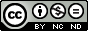### Factorization method for some inhomogeneous Lienard equations

O. Cornejo Perez, S. C. Mancas, H. C. Rosu, C. A. Rico-Olvera

#### Abstract

The factorization of inhomogeneous Li\'enard equations is performed showing that through the factorization conditions involved in the method one can obtain forcing terms for which closed-form solutions exist. Because of the reduction of order feature of factorization, the solutions are simultaneously solutions of first-order differential equations with polynomial nonlinearities. Several illustrative examples of such solutions are presented, generically having rational parts and consequently singularities.

#### Keywords

Factorization; Inhomogeneous; Li\'enard equation; Abel equation; Riccati equation

PDF

#### References

M.~Lakshmanan and S.~Rajasekar,

Nonlinear Dynamics: Integrability, Chaos, and Patterns, Springer, Heidelberg 2003.

T.~Harko and S.-D.~Liang,

Exact solutions of the Li'enard and generalized Li'enard type ordinary nonlinear differential equations obtained by

deforming the phase space coordinates of the linear harmonic oscillator,

J. Eng. Math. {bf 98} (2016) 93-111.

B.~van der Pol and J.~van der Mark, Frequency demultiplication, Nature {bf 120} (1927) 363–364.

H.C.~Rosu and O. Cornejo-P'erez,

Supersymmetric pairing of kinks for polynomial nonlinearities,

Phys. Rev. E {bf 71} (2005) 046607.

O.~Cornejo-P'erez and H.C.~Rosu,

Nonlinear second order ode's: Factorizations and particular solutions,

Prog. Theor. Phys. {bf 114} (2006) 533-538.

H.C. Rosu, O. Cornejo-P'erez, M. P'erez-Maldonado, J.A. Belinch'on,

Extension of a factorization method of nonlinear second order ODE's with variable coefficients,

Rev. Mex. F'{i}s. {bf 63} (2017) 218-222.

D.S.~Wang and H.~Li,

Single and multi-solitary wave solutions to a class of nonlinear evolution equations,

J. Math. Anal. Appl. {bf 343} (2008) 273-298.

V.K.~Chandrasekar, M.~Senthilvelan, and M.~Lakshmanan,

New aspects of integrability of force-free Duffing–van der Pol oscillator and related nonlinear systems,

J. Phys. A: Math. Gen. {bf 37} (2004) 4527-4534.

H.~Sarafian,

A closed form solution to a special normal form of Riccati equation,

Advances in Pure Mathematics {bf 1} (2011) 295

T.M.~Dunster,

Asymptotic solutions of inhomogeneous differential equations having a turning point,

Stud. Appl. Math. (2020) 1–37. %(2020) arXiv:2005.08152.

DOI: https://doi.org/10.31349/RevMexFis.67.443

### Refbacks

• There are currently no refbacks.